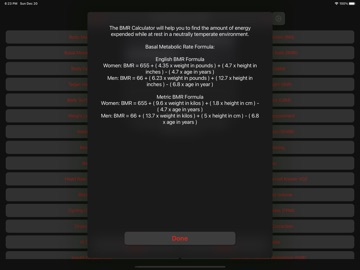Updating

### iPhone Screenshots### Description

A simple App that includes 34 Health Calculators:
- Body Mass Index (BMI) Calculator
- Body Adiposity Index (BAI) Calculator
- Basal Metabolic Rate (BMR) Calculator
- Resting Metabolic Rate (RMR) Calculator
- Body Fat Percentage Calculator
- Calorie Calculator
- Target Heart Rate (THR) Calculator
- Ideal Body Weight (IBW) Calculator
- Body Surface Area (BSA) Calculator
- Lean Body Mass (LBM) Calculator
- Weight Loss Percentage Calculator
- Human Water Requirement Calculator
- Waist Hip Ratio Calculator
- Waist Height Ratio (WHtR) Calculator
- Bench Press Calculator
- Cost of Smoking Calculator
- Beep Test Calculator
- VO2 Max Calculator
- Heart Rate Calories Burned Calculator
- Heart Rate Calories Burned Known VO2 Calculator
- Blood Volume Calculator
- Estimated Blood Volume Calculator
- Cycling Calories Burned Calculator
- Fat Free Mass Index (FFMI) Calculator
- Drops Per Minute Calculator
- Hyponatremia Correction Calculator
- IV Drip Rate Calculator
- IV Flow Rate Calculator
- Insulin Dosage Level Calculator
- Systematic Vascular Resistance (SVR) Calculator
- Weight Watchers Daily Points Plus Allowance Calculator
- Pro Points Weight Watchers Calculator
- Weight Watchers Points Plus Calculator
- Weight Watchers Old WW Points Calculator
It Also Includes:
- Description and formula for each calculator
- Clear Button
- Copy Button

### What's New in Version 3.1

- New icon
- Minor fixes and improvements

loay setrak

### Information

3.1

1.3 MB

No

Yes

Rated: 4+

#### Compatibility

iOS 13.0 or later

English

No rating

No Entry